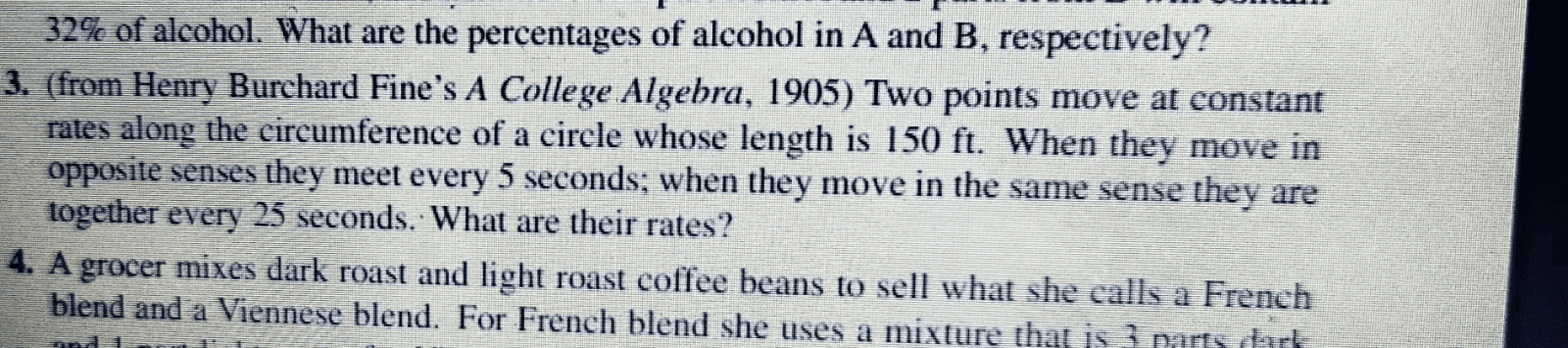# Calculating rates of two points moving along a circle

• MHB
DaalChawalQuestion 3

skeeter
Let $v_1 > v_2$ be the respective speeds (rates) in feet per second of the two particles.

Moving in opposite directions, their rate of closure is $(v_1+v_2)$

Moving in the same direction, their rate of opening is $(v_1-v_2)$

Set up a system of two equations and solve for both speeds.

Last edited by a moderator:
DaalChawal
You have added them like they are moving on straight line...In circle velocity changes as direction is changing.

skeeter
You have added them like they are moving on straight line...In circle velocity changes as direction is changing.

If the two particles move in opposite directions, the sum of their respective distances traveled in 5 seconds will be one full circumference length when they meet again.

If the two particles move in the same direction, the faster particle will move ahead of the slower particle, hence the difference between their respective distances traveled in 25 seconds will be one full circumference length when they meet again.

Using this method, I get $v_1 = 18 \, ft/sec$ and $v_2 = 12 \, ft/sec$. You can check the results yourself.

HOI
This problem has nothing to do "velocity". The problem asks for their speeds, not their velocities.